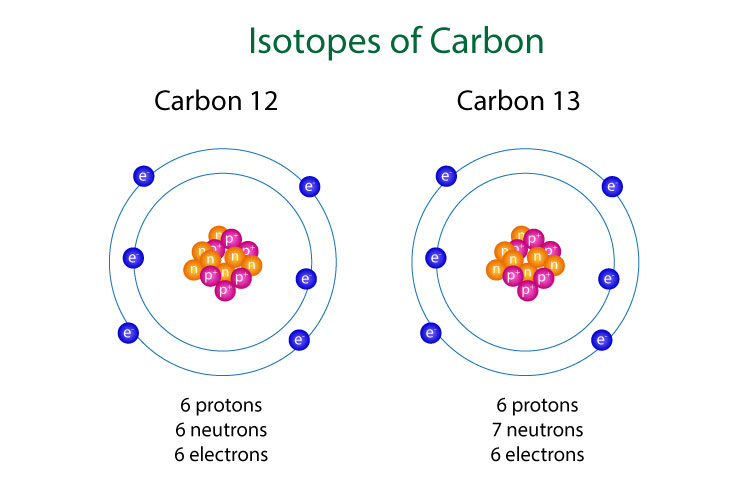# What are isotopes and how do they differ from each other?

sotopes are different forms of the same element. They have the same proton (and electron) numbers, but different neutron (and mass) numbers. Since the number of electrons of isotopes are the same, the chemical properties are also the same, as chemical reactions require the transfer/sharing of electrons. However, the physical properties of isotopes differ, since the mass numbers are different, that means the melting/boiling points and density of isotopes are also different.

Let’s investigate the isotopes of carbon: 12C and 13

Since we know isotopes have the same proton and electron numbers, we can find the number of protons of both carbon isotopes using the Periodic Table. We can then find the neutron number by subtracting the proton number from the mass number:

Protons + Neutrons = Mass Number

Neutrons = Mass Number - Protons

 Mass Number Proton Number Electron Number Neutron Number 12C 12 6 6 6 13C 13 6 6 7

Thus, from the table we can clearly see that the proton and electron numbers of the two isotopes are the same, but they differ in the neutron, hence, mass numbers.